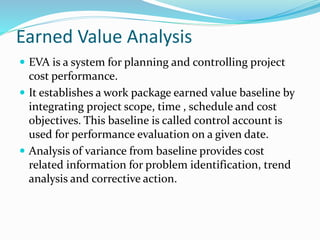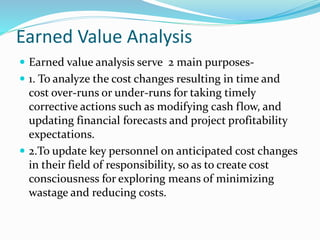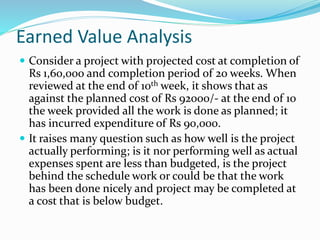Se está descargando tu SlideShare. ×

# Earned value analysis (1)

Anuncio
Anuncio
Anuncio
Anuncio
Anuncio
Anuncio
Anuncio
Anuncio
Anuncio
Anuncio
AnuncioCargando en…3
×

1 de 18 Anuncio

# Earned value analysis (1)

Earned value management Under construction project management and finance

Earned value management Under construction project management and finance

Anuncio
Anuncio

### Earned value analysis (1)

1. 1. Earned Value Analysis  EVA is a system for planning and controlling project cost performance.  It establishes a work package earned value baseline by integrating project scope, time , schedule and cost objectives. This baseline is called control account is used for performance evaluation on a given date.  Analysis of variance from baseline provides cost related information for problem identification, trend analysis and corrective action.
2. 2. Earned Value Analysis  Earned value analysis serve 2 main purposes-  1. To analyze the cost changes resulting in time and cost over-runs or under-runs for taking timely corrective actions such as modifying cash flow, and updating financial forecasts and project profitability expectations.  2.To update key personnel on anticipated cost changes in their field of responsibility, so as to create cost consciousness for exploring means of minimizing wastage and reducing costs.
3. 3. Earned Value Analysis  Consider a project with projected cost at completion of Rs 1,60,000 and completion period of 20 weeks. When reviewed at the end of 10th week, it shows that as against the planned cost of Rs 92000/- at the end of 10 the week provided all the work is done as planned; it has incurred expenditure of Rs 90,000.  It raises many question such as how well is the project actually performing; is it nor performing well as actual expenses spent are less than budgeted, is the project behind the schedule work or could be that the work has been done nicely and project may be completed at a cost that is below budget.
4. 4. Earned Value Analysis  Analysis of variance from baseline using EVA yields variety of variances that are analyzed to determine the current status, initiate corrective actions and forecast the future trends.  EVM is the process of measuring performance of project work against a baseline plan. The application of EVM enables better integration of cost and time schedule control with responsibility defined in OBS.  It provides better overall picture of performance and gives better forecast of the final costs at completion.  Thus EV represents a translation of project cost into work progress.
5. 5. Earned Value Analysis  Planned Value(PV): It is the cumulative planned value of the authorized work, scheduled for completion to date at contract rate. It represents time- phased cost projections of planned value in the budget. For example, if a project has budget of Rs 1,00,000 and 5 month schedule shows that 40 % of the value of project work should be completed at the end oof 5th month, then PV at the end of 5th month is Rs 4,00,000/-. The planned value is also called as Budgeted Cost of Work Scheduled(BCWS).
6. 6. Earned Value Analysis  Earned Value (EV) : It is the cumulative value of the authorized work completed to date at contract rate.  For example, if a project has budget of Rs 1,00,000 and work completed to date represents 25 % of the value of entire project work as against the 40 % planned, then EV at the end of 5th month is Rs 2,50,000/-. The Earned value is also called as Budgeted Cost of Work Performed.(BCWP).
7. 7. Earned Value Analysis  Actual Cost (AC) : It is the cumulative actual expenses including apportioned management reserve incurred in the project to date..  For example, if a project has budget of Rs 10,00,000 and Rs 5,00,000 has been spent on the project to date, the AC of the project would be Rs 5,00,000 . Actual Cost is also known as Actual Cost of Work Performed.(ACWP).
8. 8. Earned Value Analysis  If a project progresses exactly as per cost and time integrated plan, the 3 parameters will be identical. But this rarely happens.  The difference (EV-AC) and (EV-PV) during implementation phases generates variances.  Cost Variance: Cost Variance is computed by comparing actual performance (AC) with the budgeted cost of work performed (EV).  Cost Variance(CV)= EV-AC.  =2,50,000-500000  =-250000/-  If CV is positive the project has under-run, i.e. the cost incurred is less than planned or budgeted cost.  If CV is negative the project has over-run, i.e. the cost incurred is more than planned or budgeted cost.  If CV is zero, then the project is proceeding according to the budgeted cost.
9. 9. Earned Value Analysis  Schedule Variance(SV): Schedule Variance is computed by comparing planned performance (PV) with the budgeted cost of work performed (EV).  Schedule Variance(SV)= EV-PV.  =250000-400000  =-150000  If SV is positive then the project is ahead of its planned cost or planned schedule, i.e. earned value of the work performed is higher than planned or scheduled earned value.  If SV is negative, then the project is behind its planned cost or planned schedule, i.e. earned value of the work performed is less than planned or scheduled earned value.  If SV is zero, then the project is proceeding according to the planned schedule.
10. 10. Earned Value Analysis  Performance Indices:- It determines how efficiently the task was done and what its implications will be on future trends. The future trends in productivity, cost and time performance can be predicted as under  Cost Performance Index (CPI)= EV/AC  =250000/500000  =0.5  Schedule Performance Index(SPI)=EV/PV  =250000/400000  =0.625  An index of 1.0 or greater indicates a favorable performance and less than 1.0 implies an unfavorable trend.
11. 11. Earned Value Analysis  Estimate at completion- The project manager should prepare a to-complete forecast, which is an estimate of time and cost needed to complete the project.  This forecast plus the current status of the project provide a forecast of the date and cost of the project at completion.  Estimated Cost to complete the project(ETC) =(BAC-EV)/CPI  Where BAC is the budgeted cost at completion for the project (the same as the total planned value as of the target completion date)  ETC= (1000000-250000)/0.5  = 1500000/-
12. 12. Earned Value Analysis  EAC (Estimated cost at completion)=AC+ ETC  =500000+1500000  =2000000
13. 13. Earned Value Analysis
14. 14. Problem from tutorial
15. 15. Solution  1.Planned Value(BCWS)= 4 robots x 100000  = Rs 400000 /-  2.Earned Value (BCWP)=3 robots x 100000  =Rs 300000/-  3.Actual Cost(ACWP) =3 robots x 150000  =Rs 450000  4.Cost Variance(CV)= EV-AC  = 300000-450000  =-150000  The project has overrun by Rs 150000 till date
16. 16. Solution  5. Cost Performance Index (CPI)= EV/AC  =300000/450000  =2/3=0.667  6. Schedule Variance(SV)= EV-PV.  =300000-400000  =-100000  The project is behind schedule by Rs 100000 work  7. Schedule Performance Index(SPI)=EV/PV  =300000/400000  =0.75  The project is behind schedule by 25 % time.
17. 17. Solution  8. Estimated Cost to complete the project(ETC) =(BAC-EV)/CPI  =(400000-300000)/0.667  =Rs 150000  The project will require additional Rs 150000 to complete.  9. EAC (Estimated cost at completion)=AC+ ETC  =450000+150000  =Rs 600000  The estimated project cost will be Rs 600000 as against the budgeted cost of Rs 400000 if it continues in the same way.